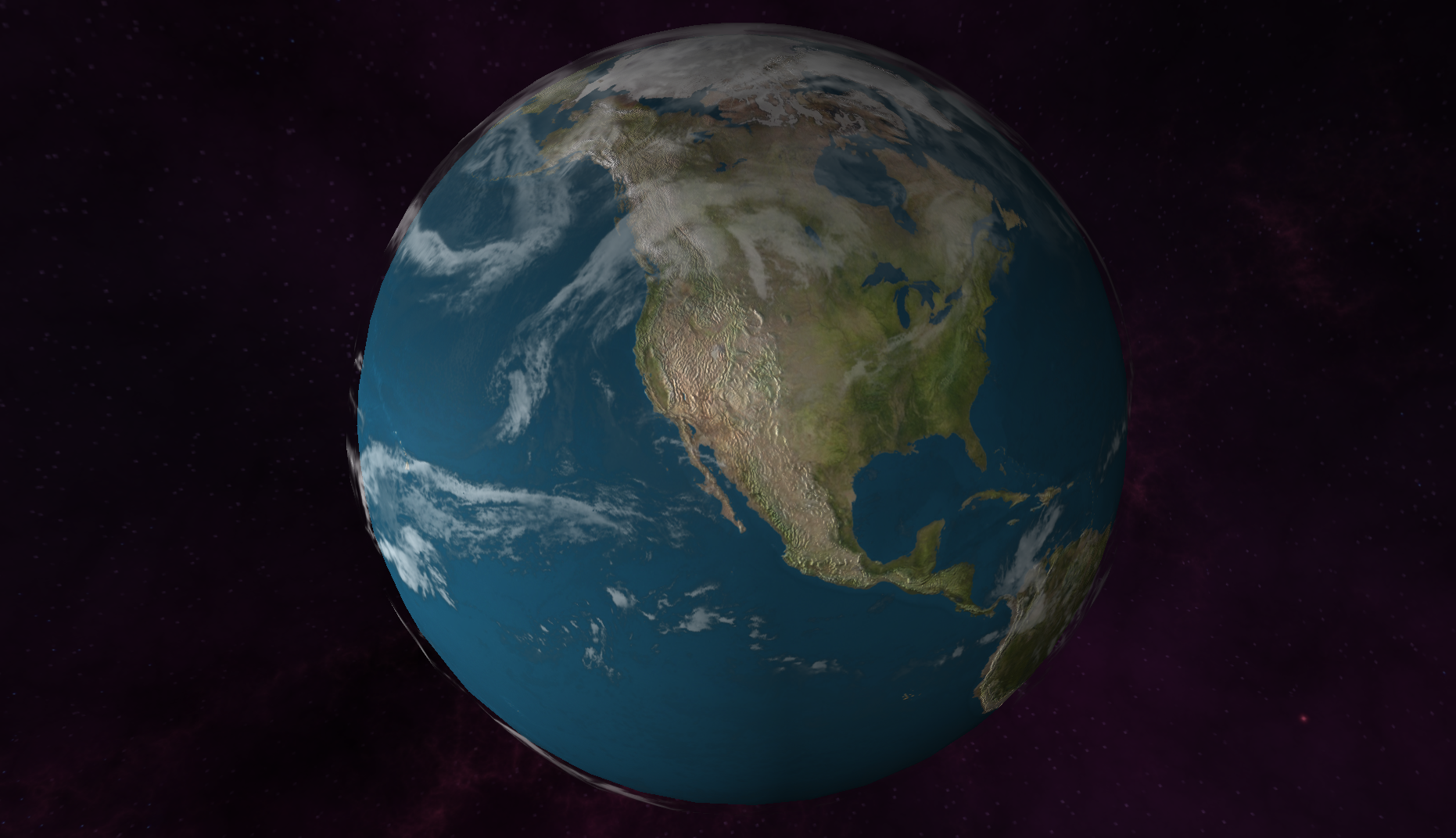### 3D Components with AngularJs

Created by Aysegul Yonet / @AysegulYonet

Slides: bit.ly/wu-threejs-angular

AnnieCannons.com

• Three.js
• AngularJS
• Three.js + AngularJS = 3D components

Tweet Migration### Why Three.js?

• Faster to write
• Faster to learn
• It is fast

Arms Globe

### 3D scene with three.JS

• Scene
• Camera
• Renderer
``````

var scene = new THREE.Scene();

``````

### Camera

``````
//FoV, AR, NC, FC
var camera = new THREE.PerspectiveCamera( 75, window.innerWidth / window.innerHeight, 0.1, 1000 );

``````

### Renderer

``````

var renderer = new THREE.WebGLRenderer();
renderer.setSize( window.innerWidth, window.innerHeight );
document.body.appendChild( renderer.domElement );

``````

``````
var scene = new THREE.Scene();
var camera = new THREE.PerspectiveCamera( 75, window.innerWidth / window.innerHeight, 0.1, 1000 );

var renderer = new THREE.WebGLRenderer();
renderer.setSize( window.innerWidth, window.innerHeight );
document.body.appendChild( renderer.domElement );
``````

• Geometry
• Material
• Mesh

### Geometry

``````
//width, height, depth
var geometry = new THREE.BoxGeometry( 1, 1, 1 );
``````

Source Code

### Material

``````
var material = new THREE.MeshBasicMaterial( { color: 0x00ff00 } );
``````

### Mesh

``````
var cube = new THREE.Mesh( geometry, material );
camera.position.z = 5;
``````

``````
var geometry = new THREE.BoxGeometry( 1, 1, 1 );
var material = new THREE.MeshBasicMaterial( { color: 0x00ff00 } );
var cube = new THREE.Mesh( geometry, material );

camera.position.z = 5;
``````

### Rendering

``````
function render() {
requestAnimationFrame( render );
renderer.render( scene, camera );
}
render();
``````

### Animation

``````
cube.rotation.x += 0.01;
cube.rotation.y += 0.01;
``````

### Group

``````
var group = new THREE.Object3D;
group.rotation.y += 0.1;
``````

### What is AngularJS

• Controllers
• Directives
• Services

``````

1 + 2 = {{ 1 + 2 }}

``````

``````

Hello {{name}}

``````

jsFiddle

### Controllers

``````

``````

``````
var app = angular.module("firstApp", []);
app.controller("MainController", function(\$scope){
//initial value
\$scope.meaning = 42;

\$scope.changeMeaning = function(){
\$scope.meaning = Math.floor(Math.random() * 42);
};
})
``````

jsFiddle

### Directives

``````
angular.module('myApp', [])
.directive('globe', function() {
return {
restrict: 'E',
}
});
``````

``````

``````
``````
angular.module('myApp', [])
.controller('MainController', function(\$scope){
\$scope.world = {name: 'earth'};

})
.directive('globe', function() {
return {
restrict: 'E',
scope: {
globe: '=info'
},
template:'Hello, {{globe.name}}'
}
})
``````

jsFiddle

``````
angular.module('myApp', [])
.directive('globe', function() {
return {
restrict: 'E',
scope: {
globe: '=info'
},
templateUrl:'templates/globe.html',
//component functionality goes here...
}
}
})
``````

#### Example Repo

Follow the steps from branch step-0 to step-5

### Angular 2

``````
@Component({selector: 'globe'})
@View({template: 'Hi {{ word.name }}'})
class MyAppComponent {
constructor() {
this.word = {name: 'earth'};
}
}
``````